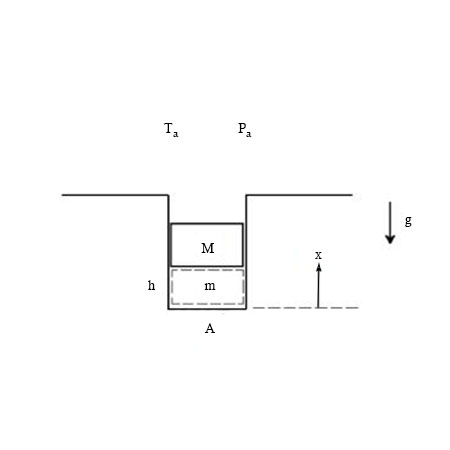# Consider a simple model of combustion inside a cavity. A cylinder of mass M can freely move up...

## Question:

Consider a simple model of combustion inside a cavity. A cylinder of mass {eq}M {/eq} can freely move up and down inside a cavity with a cross sectional area {eq}A {/eq}. The connection between the cylinder wall and the cavity wall is airtight. Below the cylinder lies an air cavity, which contains an air mass {eq}m {/eq}. Initially, the entire system is in thermal and mechanical equilibrium at the ambient temperature {eq}T_a {/eq}. The air within the cavity is then suddenly heated to a temperature {eq}T_{hot} {/eq}. During this initial heating the cylinder does not move.

a) Show that the pressure in the air cavity immediately following the initial heating is {eq}P= (P_a + \frac {Mg}{A}) \frac {T_{hot}} {T_a} {/eq}

b) Assuming that there is no friction between the cylinder and the cavity wall and that the cavity and cylinder are insulated, show that the position x, of a slowly moving cylinder satisfies the following differential equation (where h is the initial position of the bottom of the cylinder): {eq}\frac {T_{hot}}{T_a} ( P_a + \frac {Mg}{A}) (\frac {h} {x}) ^{\gamma} A-{P_a}{A} -Mg = M \frac {d^2x}{dt^2} {/eq}

c) Show that the final equilibrium position of the cylinder is {eq}x = (\frac {T_{hot}}{T_a})^{1/{\gamma}} h {/eq}

d) Is this process reversible (yes or no)? Explain why>## Reversible Process:

The process where the path can be retraced back to its initial condition, this process is known to be reversible process. For a thermodynamic process to be reversible the work done in the process should be zero.

## Answer and Explanation: 1

Become a Study.com member to unlock this answer! Create your account

(a) Initial pressure for the given figure can be known by free body diagram

So, initial pressure is

{eq}P_a+\frac{mg}{A} {/eq}

Also from Ideal gas...

See full answer below.

#### Learn more about this topic:What is Static Pressure? - Definition & Formula

from

Chapter 5 / Lesson 3
74K

Static pressure is the pressure that a fluid exerts when it is not moving. This lesson looks at the definition of static pressure as well as how to compute the pressure.